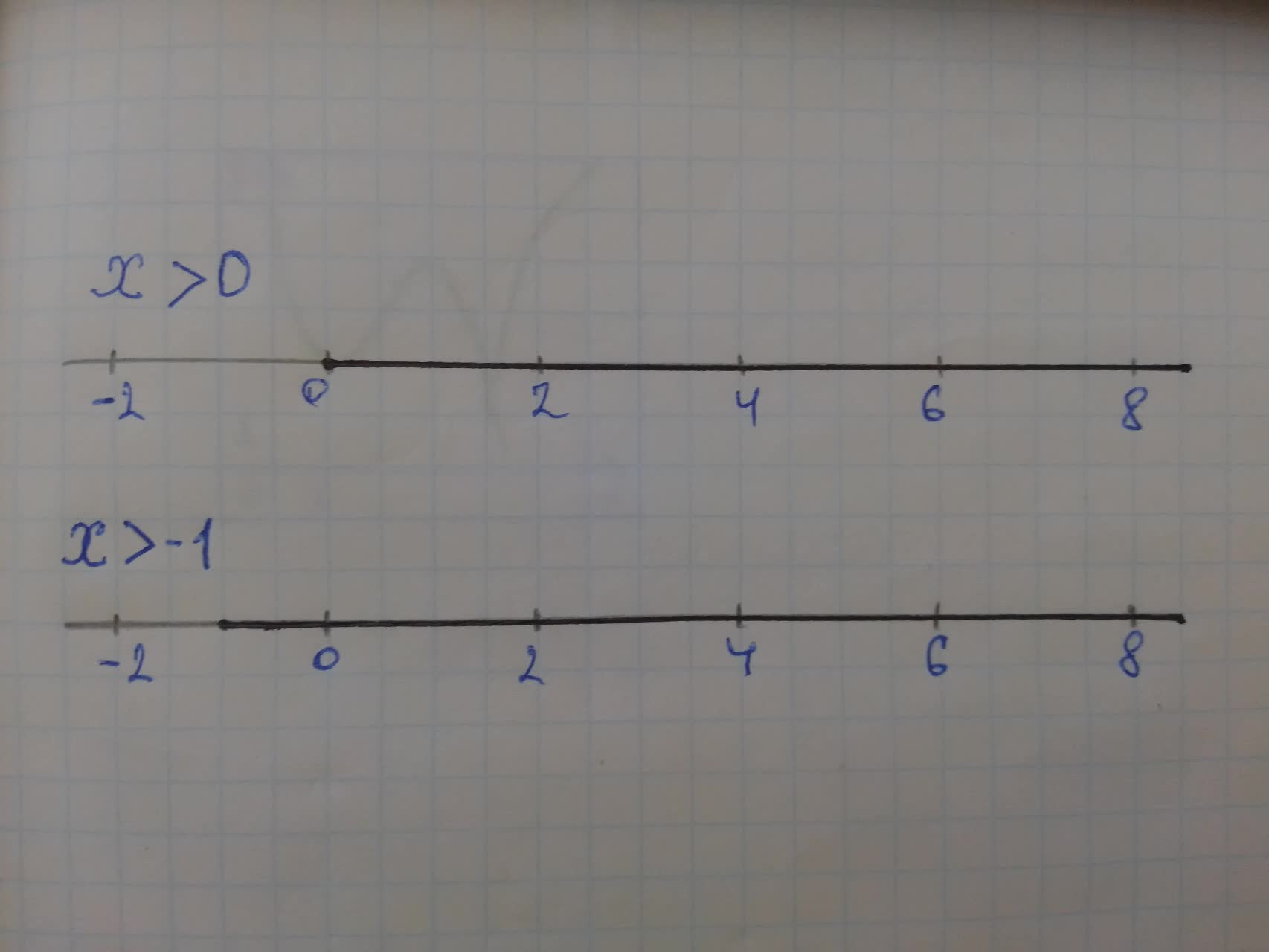# Graph the solution set for each compound inequality, and express the solution sekhi1la2f1qv 2021-11-16 Answered
Graph the solution set for each compound inequality, and express the solution sets in interval notation. $x>0$ and $x\succ 1$
You can still ask an expert for help

• Questions are typically answered in as fast as 30 minutes

Solve your problem for the price of one coffee

• Math expert for every subject
• Pay only if we can solve itAntum1978
Step 1
The given compound inequality is,
$x>0\cdots \cdots \left(i\right)$
$x\succ 1\cdots \cdots \left(i\right)$
To find the solution of the compound inequality, we find the common set of solutions which satisfies both the inequality.
For the first inequality, the value of x should be greater than 0 and for the second inequality, the value of x should be -1.
If we take a value of x as greater 0. then it will satisfy both the equations.
Step 2
Hence, the graph of the two inequalities are as,Step 3
Therefore, the required solution set is as,
$x\in \left(0,\mathrm{\infty }\right)$